# Background

This vignette aims to demonstrate the workflows used to perform contact analysis using the wildlifeDI package in R. Specifically, two datasets are used to show how the different functions for contact analysis can be used. The main contact analysis functions in the wildilfeDI package have all been given a ‘con’ prefix (e.g., conProcess) so that they clearly stand apart from the dynamic interaction indices and other functions available in the package.

# Set Up

## Libraries and Packages

library(wildlifeDI)
library(adehabitatLT)
library(ggplot2)
library(sf)

# Contact Analysis - Doe Deer Data

First let’s take a look at the doe deer data.

data(does)
does
##
## *********** List of class ltraj ***********
##
## Type of the traject: Type II (time recorded)
## * Time zone unspecified: dates printed in user time zone *
## Irregular traject. Variable time lag between two locs
##
## Characteristics of the bursts:
##            id       burst nb.reloc NAs          date.begin            date.end
## 1 d16241y2011 d16241y2011     1486   0 2011-04-30 19:02:37 2011-05-31 18:32:39
## 2 d16243y2011 d16243y2011     1480   0 2011-04-30 19:02:40 2011-05-31 18:32:37
## 3 d16244y2011 d16244y2011     1474   0 2011-04-30 19:02:36 2011-05-31 18:32:39
## 4 d16246y2011 d16246y2011     1481   0 2011-04-30 19:02:36 2011-05-31 18:32:40
## 5 d16247y2011 d16247y2011     1482   0 2011-04-30 19:02:37 2011-05-31 18:32:40
## 6 d16250y2011 d16250y2011     1474   0 2011-04-30 19:02:37 2011-05-31 18:32:37
## 7 d16252y2011 d16252y2011     1487   0 2011-04-30 19:02:40 2011-05-31 18:32:36
##
##
##  infolocs provided. The following variables are available:
##  "Elev"    "Slope"   "pForest" "dPond"   "dRoads"
plot(does)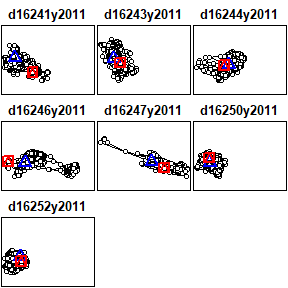## Processing contacts

We use the conProcess function to identify contacts first. We use a temporal threshold of $$t_c$$ = 15 minutes (based on the 30 minute tracking data) to define simultaneous fixes. A distance threshold of $$d_c$$ = 50 m (based on biologically relevant signals between deer and previous literature) was used to define contacts. The parameter dc must be specified in the correct units (i.e., those associated with the tracking dataset). The parameter tc needs to be specified in seconds. We can look at the distribution of all paired fixes (based on tc) to further explore whether our choice for dc makes sense.

plt <- dcPlot(does,tc=15*60,dmax=1000)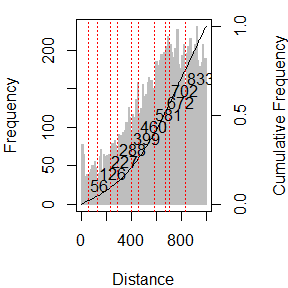plt
##    55.55449 126.26021 227.26837 287.87327 398.98226 459.58716 580.79696
##   671.70431 702.00676 833.31737

The red lines in the dcPlot are automatically generated using a natural breaks algorithm to find local minima. They are more for reference, and not to be used for empirical assessment. That being said, it appears that a choice of $$d_c$$=50 corresponds to the first local minima.

doecons <- conProcess(does,dc=50,tc=15*60)  

Next we can arrange contacts between does into phases of continuous interaction using the function conPhase. A parameter $$p_c$$ is used to group contacts as belonging to the same phases seperated by pc units in time. The parameter pc must be specified in seconds. The function conSummary can be used to summarize contacts and phases within the entire dataset to get some basic statistics. It computes how many contacts are observed, and in how many unique segments these occur in, as well as some other values regarding contact phase durations. Here $$p_c$$ = 60 minutes.

doephas <- conPhase(doecons, pc=60*60)
conSummary(doephas)
##                   stat result
## 1              n Fixes  10364
## 2           n Contacts    493
## 3             n Phases     99
## 4 Longest Phase (secs)  66574
## 5    Mean Phase (secs)   8154
## 6  Median Phase (secs)   3601
## 7   no. one-fix Phases     33

The summary stats suggest contacts between does are often over short bursts, but in some cases can be continuous over much longer periods of time.

## Temporal Analysis of Contacts

The conPairs and conTemporal functions can be used to extract more detailed information about the timing and duration of phases within the dataset. We plot the frequency histogram of contacts throughout the day (by hour), then the histogram of contacts throughout the month of May (by day), and the histogram of the duration of all contact phases.

doepair <- conPairs(doephas)
doetemp <- conTemporal(doephas,units='mins')

doepair$hod <- as.POSIXlt(doepair$date)$hour + as.POSIXlt(doepair$date)$min / 60 #convert POSIX to hours doetemp$hod <- as.POSIXlt(doetemp$start_time)$hour + as.POSIXlt(doetemp$start_time)$min / 60  #convert POSIX to hours
doepair$dom <- as.POSIXlt(doepair$date)$mday hist(doepair$dom,breaks=0:31)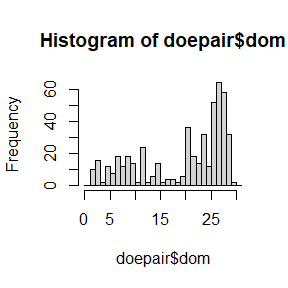We can see a clear cluster of contacts occurring near the end of the month, this is likely associated with parturition cycles, and the onset of denning behaviour.

hist(doepair$hod,breaks=0:24) #Figure 2b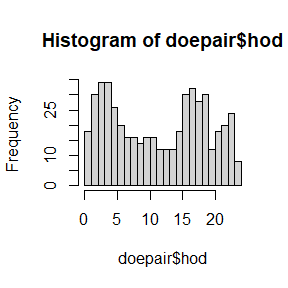We can see the clear diurnal pattern in when contacts occur, which corresponds to known activity peaks in deer. There is a curious minimum occurrig at approximately 4-5 am right before the morning activity peak. hist(doetemp$hod,breaks=0:24) #Figure 2c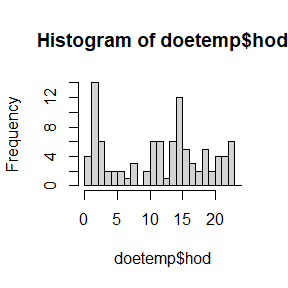Again a similar pattern emerges when we only look at the timing of the initiation of contact phases. Finally, we can look at the distribution of the duration of the contact phases.

hist(as.numeric(doetemp\$duration)) #figure 2d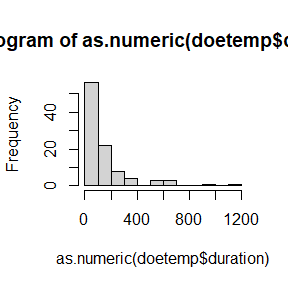### Mapping Contacts

Using the conSpatial function and the parameter choices we can easily make maps of contacts. First plot the distribution of all contact points on top of the distribution of all GPS fixes.

con_sf <- conSpatial(doephas,type='point')             # Get points of all contacts

#Figure 3a
sf_pt <- ltraj2sf(does)  # Turn all fixes into sf points
plot(st_geometry(sf_pt),col='grey',pch=20)
plot(st_geometry(con_sf),col='black',pch=20,add=T)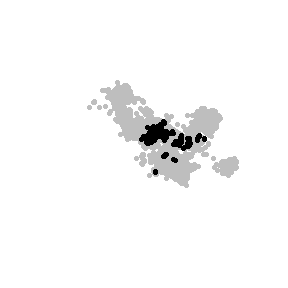We can see that the contacts are clustered around certain locations when compared to all GPS telemetry fixes.

Next, lets map only the initiation of phases (i.e., the first fix in every phase).

#Figure 3b
con_sf_first <- conSpatial(doephas,type='point',def='first')

plot(st_geometry(sf_pt),col='grey',pch=20)
plot(st_geometry(con_sf),col='black',pch=20,add=T)
plot(st_geometry(con_sf_first),col='red',pch=20,add=T)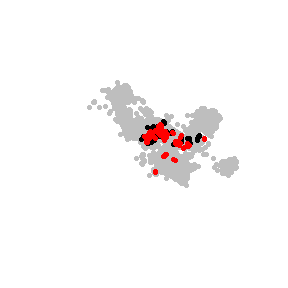Here we can see a difference in the spatial pattern of the initiation of contact phases the original distribution of GPS fixes, and the locations of all contact points.

Finally, lets map the contact phases as lines.

#Figure 3c
con_sf_ln <- conSpatial(doephas,type='line')

sf_ln <- ltraj2sf(does,type='line')  # Turn all fixes into sf points

plot(st_geometry(sf_ln),col='grey')
plot(st_geometry(con_sf_ln),col='red',add=T)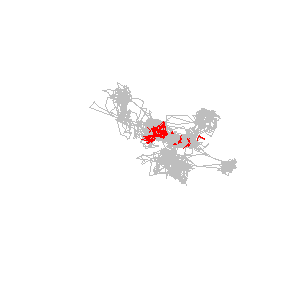The map of the phases as lines provides different insight into the spatial structure of contact phases throughout the study area.

# Contact Analysis - Mock Hunters and Deer Bucks

The initial steps of contact analysis are very similar to the contact analysis for the does. Here, instead of providing the raw data (which was too large) we provide the data processed after running the function conContext. The steps are shown below but not run. The parameters are defined as specified in the manuscript, and finally we chose three contextual/behavioural variables to explore: step length (termed dist), displacement from contact, and percent forest cover.

# NOT RUN
mca <- conProcess(deer,hunters,dc=150,tc=4*60) # process contacts, tc=4 min, dc=150m
mcp <- conPhase(mca,pc=16*60)                  # group into phases pc=16 min

mcp <- conDisplacement(mcp,contact='first')    # calculate displacement

#Context Analysis
mockhunt <- conContext(mcp,var=c('dist','displacement','Forest_Perc'),contact='first',
nlag=12,lag=8*60,gap=4*60,idcol='burst',nrand=200)

We can load in these data, to take a look at the structure of this dataframe.

data(mockhunt)
head(mockhunt)
##                     date       dist displacement Forest_Perc dt_con dt_lev
## 2757 2008-12-16 15:38:37  10.440307     0.000000       26.03      0    Con
## 2756 2008-12-16 15:30:36   2.236068     2.236068       26.03   -481     B1
## 2758 2008-12-16 15:46:36   6.708204    10.440307       26.03    479     A1
## 2755 2008-12-16 15:22:36  15.524175    14.142136       26.65   -961     B2
## 2759 2008-12-16 15:54:36 365.531120     7.615773       26.03    959     A2
## 2754 2008-12-16 15:14:37   9.848858     5.385165       26.03  -1440     B3
##      phaid     ID
## 2757     1 2008_1
## 2756     1 2008_1
## 2758     1 2008_1
## 2755     1 2008_1
## 2759     1 2008_1
## 2754     1 2008_1

The output from conContext can be easily used to study before and after contact events by looking at the dt_con and dt_level columns which provide the time (in seconds) before or after the contact, and a factor ‘level’ basedon the lags that were input into the conContext function. Here we define a contact as occurring at the first fix in a contact phase. Other definitions are of course possible. Then we look at 12 x 8 minute lags before and after each contact. The four gap argument is useful to center the lags at the regular fix recording times so small deiations in the GPS times recorded are kept within the correct lag. To do this set the gap argument to 1/2 the fix interval.

There are two useful ways to look at these data:

1. a lineplot
2. a boxplot

The lineplot can be used to look at patterns that change over time individually, whereas a boxplot can be used to look at general trends grouping all the individuals together.

If we first look at the line plots for each of the three variables we can get an idea of any changes in behaviour or context before and after contact events. In such a plot, each line represents a single contact event (phase; $$n=47$$). The x-axis is the time before and after the first fix in the contact phase. The y-axis is any one of the behaviour or contextual variables; here we will first look at step-length.

ggplot(data=mockhunt, aes(x=dt_lev, y=dist, group=phaid)) +
geom_line(col='grey32') +
labs(x='Time to contact (min)',y='Step-length (m)') +
scale_x_discrete(labels=c(as.character(seq(-96,96,by=8)),'R'))
## Warning: Removed 4 row(s) containing missing values (geom_path).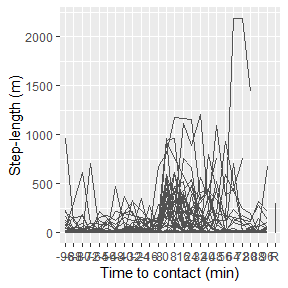Here we can see evidence of increased moment following contacts with mock-hunters, but a high degree of variation between individuals. It is unclear precisely how long after a contact with a mock-hunters the effect lasts.

We can look at the spatial displacement (e.g., actual geographic distance) a deer makes following a contact as well.

ggplot(data=mockhunt, aes(x=dt_lev, y=displacement, group=phaid)) +
geom_line(col='grey32') +
labs(x='Time to contact (min)',y='Displacement (m)') +
scale_x_discrete(labels=c(as.character(seq(-96,96,by=8)),'R'))
## Warning: Removed 1 row(s) containing missing values (geom_path).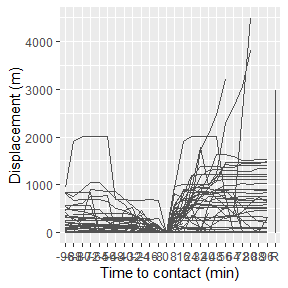In the above plot we can see some unusually high movement behaviour by one or two individuals, but also that there is clearly larger displacement away from contact locations after contacts compared to before.

Last, we can look at how animals use forest cover before and after contacts.

ggplot(data=mockhunt, aes(x=dt_lev, y=Forest_Perc, group=phaid)) +
geom_line(col='grey32') +
labs(x='Time to contact (min)',y='Forest Cover (%)') +
scale_x_discrete(labels=c(as.character(seq(-96,96,by=8)),'R'))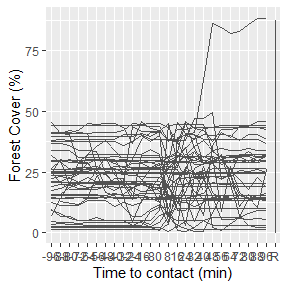From this analysis it is less clear how forest coverage is used by deer before and after contacts. It appears that the increased movement behaviour shown through step-length and displacement may result in changes in forest coverage levels. However, it does not appear that deer prefer or avoid forest coverage in response to contacts from hunters based on these data.

A perhaps cleaner way to look at these same patterns is to use boxplots.

ggplot(mockhunt, aes(x=dt_lev, y=dist)) +
geom_boxplot() +
coord_cartesian(ylim=c(0,1000)) +
labs(x='Time to contact (min)',y='Step length (m)') +
scale_x_discrete(labels=c(as.character(seq(-96,96,by=8)),'R'))
## Warning: Removed 4 rows containing non-finite values (stat_boxplot).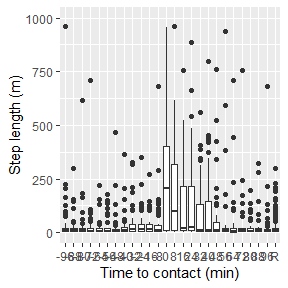From this boxplot we can see the clear pattern of increased movement after contact events. It appears based on this boxplot that the effect of the contact manifests in increased movement (step lengths) for about $$6 \times 8 = 48$$ minutes post contact.

Next, we will look at the displacement (distance-to-contact) effect using the box plots.

ggplot(mockhunt, aes(x=dt_lev, y=displacement)) +
geom_boxplot() +
coord_cartesian(ylim=c(0,2000)) +
labs(x='Time to contact (min)',y='Distance to contact (m)') +
scale_x_discrete(labels=c(as.character(seq(-96,96,by=8)),'R'))
## Warning: Removed 51 rows containing non-finite values (stat_boxplot).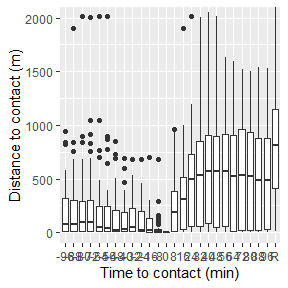In this graph, it can be clearly seen that contacts result in a spatial displcament by white-tailed deer on average about 500 m displacement from their pre-contact position. This is important information to consider, as it means that white-tailed deer typically move ~500m following contact with a human hunter. Note, the comparison with random displacements is not useful in this particular case.

Of interest is whether there are any environmental changes in the habitats chosen following contacts, here we will look at the percent forest cover as one such example.

ggplot(mockhunt, aes(x=dt_lev, y=Forest_Perc)) +
geom_boxplot() +
labs(x='Time to contact (min)',y='Forest Cover (%)') +
scale_x_discrete(labels=c(as.character(seq(-96,96,by=8)),'R'))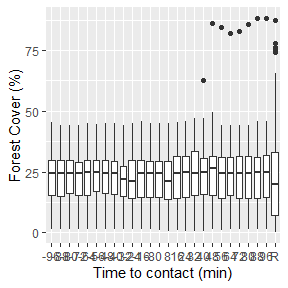The visual evidence here suggests (as noted with the line graph) that deer do not preferentially select or avoid forest habitat before, during, or after contacts. The distribution of forest habitat found before, during, and after contacts is comparable to the distribution associated with randomly chosen fixes.

# Summary

The wildlifeDI package can be used to tackle a wide range of problems when performing contact analysis using wildlife tracking data. Specifically, it provides tools to process, manage, and analyze contacts spatially and temporally. It provides output data structures that are useful for integration in R’s well established statistical modelling packages facilitating further statistical analyses.